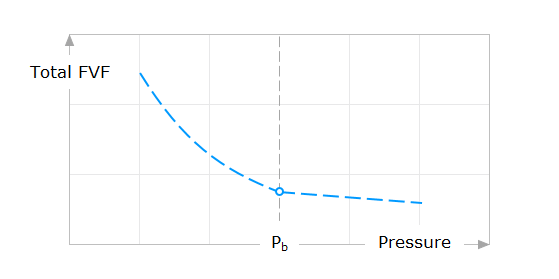# Free Online PVT Calculator

Calculate the properties of petroleum reservoir fluids with a reliable, simple PVT calculator. Use the Glaso total formation volume factor calculator.

## Total Formation Volume Factor

Glaso (1980)

First-stage separator gas specific gravity
Stock-tank oil specific gravity
Solution gas-oil ratio at bubble point pressure

N.B. The total (two-phase) formation volume factor equation is used along with the Dranchuk-Abou-Kassem (DAK) (1975) correlation for the gas formation volume factor and the Glaso (1980) correlation for the oil formation volume factor.

Want more details? Discover Glaso (1980) correlations.

## Fluid Property Behavior

### Total Formation Volume Factor

In this section, I would like to make sure that you can easily describe the behavior of the total (two-phase) formation volume factor property at any reservoir pressures, whether the pressure is less than or greater than the oil bubble point pressure. Therefore, I will give you brief technical notes by answering three questions.Behavior of the total FVF versus pressure
Why does the total formation volume factor increase as the pressure decreases in the reservoir?
At pressures greater than bubble point pressure, the total (two-phase) formation volume factor increases gently as the reservoir pressure decreases. That behavior occurs because the oil phase, holding the maximum amount of gas in solution, expands gently due to the pressure decrease. When the pressure decreases below the bubble point pressure, the total (two-phase) formation volume factor increases rapidly.​ That’s because more gas is liberating from the liquid phase, making the whole system expanded rapidly. Actually, almost all the black oil properties respond differently to the decrease in reservoir pressure.
What are the black oil PVT properties that are affecting the value estimated for the total formation volume factor?
The total formation volume factor is strongly dependent on the values estimated for the solution gas-oil ratio, the oil formation volume factor, and the gas formation volume factor.
What are the black oil PVT properties that depend on the value estimated for the total formation volume factor?
Actually, all other black oil PVT properties are independent of the value estimated for the total formation volume factor.

## Calculate more PVT properties

Use the most powerful fluid property calculator.

## Want to calculate even more?

Try PVT Solver for free and figure out how powerful and simple it is.

Sunyani, Ghana

5 days ago

Someone just downloaded PVT Solver for free.

Verified User

It's Popular

Atyrau, Kazakhstan

4 days ago

Someone just downloaded PVT Solver for free.

Verified User

It's Popular

Lagos, Nigeria

4 days ago

Someone just downloaded PVT Solver for free.

Verified User

It's Popular

Bayern, Germany

3 days ago

Someone just downloaded PVT Solver for free.

Verified User

It's Popular

Douala, Cameron

3 days ago

Someone just downloaded PVT Solver for free.

Verified User

It's Popular